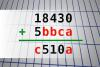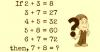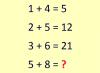BRAIN TEASERSBrain Teasers User Profile

# Ricardo Cardona

rank
506
points
3
See full ranking list
short ranking list
 504 Ine Troelstra 3 505 محمد حيدري 3 506 Ricardo Cardona 3 507 Aja Romporas 3 508 Rosʜʌŋ Zane 3
 Find number abc If 18430 + 5bbca = c510a find number abc. Multiple solutions may exist.Solve Math Puzzle If 2 + 3 = 8, 3 + 7 = 27, 4 + 5 = 32, 5 + 8 = 60, 6 + 7 = 72, then, 7 + 8 = ?Calculate 5+8 If 1+4=5, 2+5=12 and 3+6=21 then 5+8=?